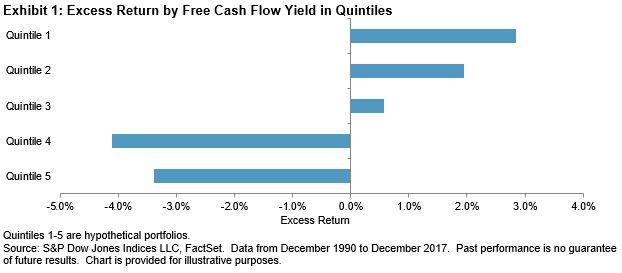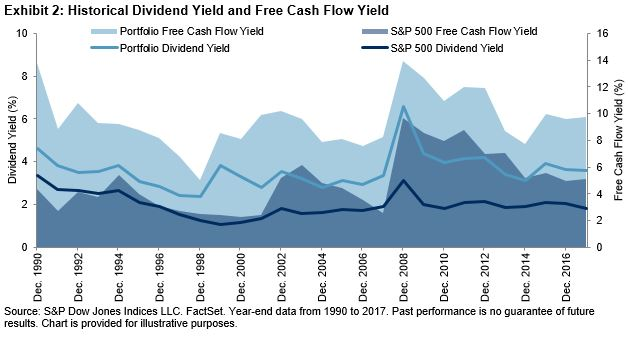# Evaluating Various Financial Ratios Used in Dividend Analysis

There are many ratios that are used to assess the strength of dividends. In this blog, we explore the commonly used dividend measures in income analysis and their applications. We also introduce cash-flow-based measurements, such as free cash flow yield, that can be combined with traditional dividend measurements to provide additional insight into the quality of dividends.

The most frequently used measure—dividend payout ratio, which is calculated as dividend per share divided by earnings per share—shows what percentage of its profit a company is returning to its shareholders in the form of cash dividends. A low dividend payout ratio means that a company is returning a small portion of its earnings to investors, while a high payout ratio implies that a company uses the majority of its profit for dividends instead of for future growth.

Dividend coverage ratio tells the same story, with the exception that the ratio is calculated as the inverse of dividend payout ratio. Therefore, a low dividend payout ratio and a high dividend coverage ratio have the same indication for the quality of the dividend. Both dividend payout ratio and dividend coverage ratio can be paired up with dividend yield information to help evaluate a company’s dividend payment capabilities. For example, a company with a high dividend yield and low dividend payout ratio (or high dividend coverage ratio) indicates that the company’s dividend yield is supported by its strong earnings.

Consistently increasing dividends is one indication that dividends are supported by a company’s earnings and the firm is confident in its ability to generate profits. That rationale is also the investment thesis behind dividend growth strategies. Dividend growth rate, another important metric in dividend analysis, shows the percentage increase in dividends and can be presented as the product of return on equity (ROE) and earnings retention rate (which is 1 minus payout ratio). The formula shows that dividend growth is influenced by a company’s profitability and dividend payout ratio. A company with a high payout ratio (therefore a low retention rate) may still result in a high dividend growth rate if payouts are supported by high profits (ROE).

While the dividend growth rate helps capture the increases in dividend income, it is often considered an unpredictable factor.  In addition, dividend yield, payout ratio, coverage ratio, and growth rate all evaluate dividends with respect to net income, which is an accrual accounting concept. Since the amount of dividends paid is shown on a company’s cash flow statement, another accepted measure is to use cash flow related fundamentals, such as free cash flow yield, to provide additional insight on company’s financial condition.

In our recently published research paper (Incorporating Free Cash Flow Yield in Dividend Analysis), we divided the S&P 500 member stocks into quintiles based on free cash flow yield. As of Dec. 31, 2017, the top-quintile stocks generated higher excess return than the remaining quintiles and outperformed the overall market by an average of 2.8% over the previous 27 years (see Exhibit 1).In the paper, we incorporated free cash flow yield into a dividend strategy. Our analysis of a combined dividend and free cash flow yield portfolio showed that a stable dividend income can be achievable when funded by sufficient free cash flow. As displayed in Exhibit 2, the portfolio’s 3.57% average dividend yield was supported by a 9.5% average free cash flow yield, compared with the benchmark’s 1.99% average dividend yield funded by 4.87% average free cash flow yield over the sampled history.Cochrane, John H., The Dog That Did Not Bark: A Defense of Return Predictability. 2008.

   A hypothetical large-cap portfolio was formed with S&P 500 sector leaders in terms of integrated multi-factor value. The integrated value is the product of dividend yield score and free cash flow yield score, each of which is computed as transforming the standardized fundamental data to cumulative normal distribution, in the range of 0 to 1.

The posts on this blog are opinions, not advice. Please read our disclaimers.